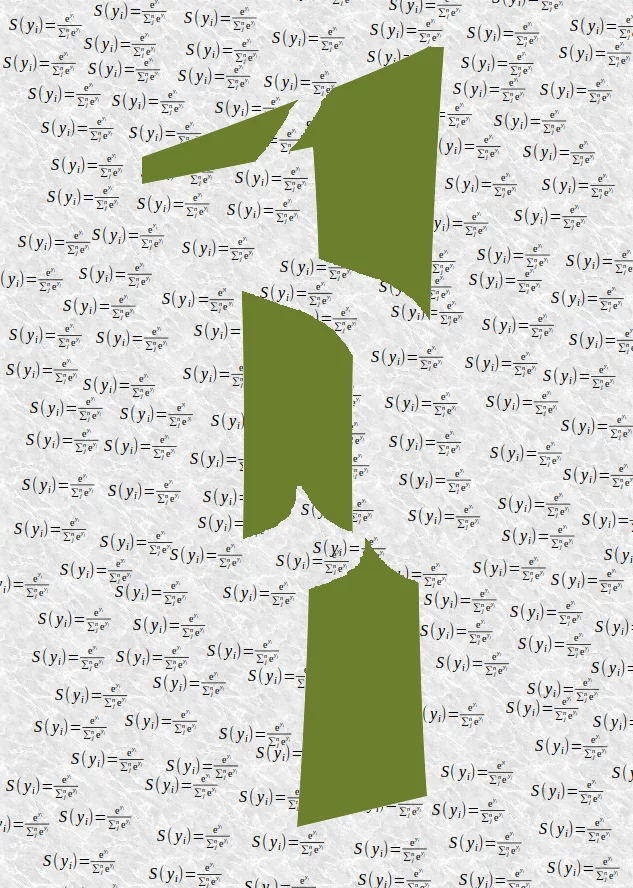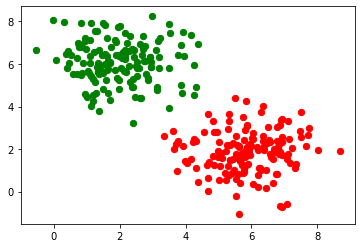## Softmax as Activation Function

### SoftmaxThe previous implementations of neural networks in our tutorial returned float values in the open interval (0, 1). To make a final decision we had to interprete the results of the output neurons. The one with the highest value is a likely candidate but we also have to see it in relation to the other results. It should be obvious that in a two classes case ($c_1$ and $c_2$) a result (0.013, 0.95) is a clear vote for the class $c_2$ but (0.73, 0.89) on the other hand is a different thing. We could say in this situation '$c_2$ is more likely than $c_1$, but $c_1$ has still a high likelihood'. Talking about likelihoods: The return values are not probabilities. It would be a lot better to have a normalized output with a probability function. Here comes the softmax function into the picture. The softmax function, also known as softargmax or normalized exponential function, is a function that takes as input a vector of n real numbers, and normalizes it into a probability distribution consisting of n probabilities proportional to the exponentials of the input vector. A probability distribution implies that the result vector sums up to 1. Needless to say, if some components of the input vector are negative or greater than one, they will be in the range (0, 1) after applying Softmax . The Softmax function is often used in neural networks, to map the results of the output layer, which is non-normalized, to a probability distribution over predicted output classes.

The softmax function $\sigma$ is defined by the following formula:

$\sigma(o_i) = \frac{e^{o_i}}{\sum_{j=1}^{n} e^{o_j}}$

where the index i is in (0, ..., n-1) and o is the output vector of the network

$o = (o_0, o_1, \ldots, o_{n-1})$

We can implement the softmax function like this:

import numpy as np

def softmax(x):
""" applies softmax to an input x"""
e_x = np.exp(x)
return e_x / e_x.sum()

x = np.array([1, 0, 3, 5])
y = softmax(x)
y, x / x.sum()

Output:
(array([0.01578405, 0.00580663, 0.11662925, 0.86178007]),
array([0.11111111, 0.        , 0.33333333, 0.55555556]))

Avoiding underflow or overflow errors due to floating point instability:

import numpy as np

def softmax(x):
""" applies softmax to an input x"""
e_x = np.exp(x - np.max(x))
return e_x / e_x.sum()

softmax(x)

Output:
array([0.01578405, 0.00580663, 0.11662925, 0.86178007])

x = np.array([0.3, 0.4, 0.00005], np.float64) print(softmax(x)) print(x / x.sum())

### Derivate of softmax Function

The softmax function can be written as $$S(o): \begin{bmatrix} o_{1}\\ o_{2}\\ \cdots\\ o_{n}\\ \end{bmatrix} \longrightarrow \begin{bmatrix} s_{1}\\ s_{2}\\ \cdots\\ s_{n}\\ \end{bmatrix}$$

Per element it looks like this:

$$s_j(o) = \frac{e^{o_j}}{\sum\limits_{k=1}^{n}{e^{o_k}}}, \forall k=1, \cdots, n$$

The derivative of softmax can be calculated like this:

$$\frac{\partial S}{\partial O} = \begin{bmatrix} \frac{\partial s_1}{\partial o_1} & \cdots & \frac{\partial s_1}{\partial o_n} \\ \cdots \\ \frac{\partial s_n}{\partial o_1} & \cdots & \frac{\partial s_n}{\partial o_n} \\ \end{bmatrix}$$

The partial derivatives can be solved for every i and j:

$$\frac{\partial s_i}{\partial o_j} = \frac{\partial \frac{e^{o_i}}{\sum_{k=1}^{n}{e^{o_k}}}}{\partial o_j}$$

We will use the quotien rule, i.e.

the derivative of

$$f(x) = \frac{g(x)}{h(x)}$$

is

$$f'(x) = \frac{g'(x)\cdot h(x) - g(x) \cdot h'(x)}{(h(x)^2}$$

We can set $g(x)$ to $e^{o_i}$ and $h(x)$ to $\sum_{k=1}^{n}{e^{o_k}}$

The derivative of $g(x)$ is

$$g'(x) = \left\{ \begin{array}{@{}ll@{}} e^{o_i}, & \text{if}\ i=j \\ 0, & \text{otherwise} \end{array}\right.$$

and the derivative of $h(x)$ is

$$h'(x) = e^{o_j}, \forall k=1, \cdots, n$$

Let's apply the quotient rule by case differentiation now:

1. case: $i = j$:
$$\frac{e^{o_i} \cdot \sum_{k=1}^{n}{e^{o_k}} - e^{o_i} \cdot e^{o_j}}{(\sum_{k=1}^{n}{e^{o_k}})^2}$$

We can rewrite this expression as

$$\frac{e^{o_i}}{\sum_{k=1}^{n}{e^{o_k}}} \cdot \frac{\sum_{k=1}^{n}{e^{o_k} - e^{o_j}}}{\sum_{k=1}^{n}{e^{o_k}}}$$

Now we can reduce the second quotient:

$$\frac{e^{o_i}}{\sum_{k=1}^{n}{e^{o_k}}} \cdot (1 - \frac{e^{o_j}}{\sum_{k=1}^{n}{e^{o_k}}})$$

If we compare this expression with the Definition of $s_i$, we can rewrite it to:

$$s_i \cdot (1 - s_j)$$

which is the same as $$s_i \cdot (1 - s_i)$$ because $i=j$.

1. case: $i \neq j$:
$$\frac{0 \cdot \sum_{k=1}^{n}{e^{o_k}} - e^{o_i} \cdot e^{o_j}}{(\sum_{k=1}^{n}{e^{o_k}})^2}$$

this can be rewritten as:

$$- \frac{e^{o_i}}{\sum_{k=1}^{n}{e^{o_k}}}\cdot \frac{e^{o_j}}{\sum_{k=1}^{n}{e^{o_k}}}$$

this gives us finally:

$$-s_i \cdot s_j$$

We can summarize these two cases and write the derivative as:

$$g'(x) = \left\{ \begin{array}{@{}ll@{}} s_i \cdot (1 - s_i), & \text{if}\ i=j \\ -s_i \cdot s_j, & \text{otherwise} \end{array}\right.$$

If we use the Kronecker delta function1, we can get rid of the case differentiation, i.e. we "let the Kronecker delta do this work":

$$\frac{\partial s_i}{\partial o_j} = s_i(\delta_{ij} - s_j)$$

Finally we can calculate the derivative of softmax:

$$\frac{\partial S}{\partial O} = \begin{bmatrix} s_1(\delta_{11} - s_1) & s_1(\delta_{12} - s_2) & \cdots & s_1(\delta_{1n} - s_n) \\ s_2(\delta_{21} - s_1) & s_2(\delta_{22} - s_2) & \cdots & s_2(\delta_{2n} - s_n) \\ \cdots \\ s_n(\delta_{n1} - s_1) & s_n(\delta_{n2} - s_2) & \cdots & s_n(\delta_{nn} - s_n) \\ \end{bmatrix}$$
import numpy as np

def softmax(x):
e_x = np.exp(x)
return e_x / e_x.sum()

s = softmax(np.array([0, 4, 5]))

si_sj = - s * s.reshape(3, 1)
print(s)
print(si_sj)
s_der = np.diag(s) + si_sj
s_der

[0.00490169 0.26762315 0.72747516]
[[-2.40265555e-05 -1.31180548e-03 -3.56585701e-03]
[-1.31180548e-03 -7.16221526e-02 -1.94689196e-01]
[-3.56585701e-03 -1.94689196e-01 -5.29220104e-01]]

Output:
array([[ 0.00487766, -0.00131181, -0.00356586],
[-0.00131181,  0.196001  , -0.1946892 ],
[-0.00356586, -0.1946892 ,  0.19825505]])
import numpy as np
from scipy.stats import truncnorm

def truncated_normal(mean=0, sd=1, low=0, upp=10):
return truncnorm(
(low - mean) / sd, (upp - mean) / sd, loc=mean, scale=sd)

@np.vectorize
def sigmoid(x):
return 1 / (1 + np.e ** -x)

def softmax(x):
e_x = np.exp(x)
return e_x / e_x.sum()

class NeuralNetwork:

def __init__(self,
no_of_in_nodes,
no_of_out_nodes,
no_of_hidden_nodes,
learning_rate,
softmax=True):
self.no_of_in_nodes = no_of_in_nodes
self.no_of_out_nodes = no_of_out_nodes
self.no_of_hidden_nodes = no_of_hidden_nodes
self.learning_rate = learning_rate
self.softmax = softmax
self.create_weight_matrices()

def create_weight_matrices(self):
""" A method to initialize the weight matrices of the neural network"""
self.weights_in_hidden = X.rvs((self.no_of_hidden_nodes,
self.no_of_in_nodes))
self.weights_hidden_out = X.rvs((self.no_of_out_nodes,
self.no_of_hidden_nodes))

def train(self, input_vector, target_vector):
"""
input_vector and target_vector can be tuples, lists or ndarrays
"""
# make sure that the vectors have the right shape
input_vector = np.array(input_vector)
input_vector = input_vector.reshape(input_vector.size, 1)
target_vector = np.array(target_vector).reshape(target_vector.size, 1)

output_vector_hidden = sigmoid(self.weights_in_hidden @ input_vector)
if self.softmax:
output_vector_network = softmax(self.weights_hidden_out @ output_vector_hidden)
else:
output_vector_network = sigmoid(self.weights_hidden_out @ output_vector_hidden)

output_error = target_vector - output_vector_network
if self.softmax:
ovn = output_vector_network.reshape(output_vector_network.size,)
si_sj = - ovn * ovn.reshape(self.no_of_out_nodes, 1)
s_der = np.diag(ovn) + si_sj
tmp =  s_der @ output_error
self.weights_hidden_out += self.learning_rate  * (tmp @ output_vector_hidden.T)
else:
tmp = output_error * output_vector_network * (1.0 - output_vector_network)
self.weights_hidden_out += self.learning_rate  * (tmp @ output_vector_hidden.T)

# calculate hidden errors:
hidden_errors = self.weights_hidden_out.T @ output_error
# update the weights:
tmp = hidden_errors * output_vector_hidden * (1.0 - output_vector_hidden)
self.weights_in_hidden += self.learning_rate * (tmp @ input_vector.T)

def run(self, input_vector):
"""
running the network with an input vector 'input_vector'.
'input_vector' can be tuple, list or ndarray
"""
# make sure that input_vector is a column vector:
input_vector = np.array(input_vector)
input_vector = input_vector.reshape(input_vector.size, 1)
input4hidden = sigmoid(self.weights_in_hidden @ input_vector)
if self.softmax:
output_vector_network = softmax(self.weights_hidden_out @ input4hidden)
else:
output_vector_network = sigmoid(self.weights_hidden_out @ input4hidden)

return output_vector_network

def evaluate(self, data, labels):
corrects, wrongs = 0, 0
for i in range(len(data)):
res = self.run(data[i])
res_max = res.argmax()
if res_max == labels[i]:
corrects += 1
else:
wrongs += 1
return corrects, wrongs

from sklearn.datasets import make_blobs

n_samples = 300
samples, labels = make_blobs(n_samples=n_samples,
centers=([2, 6], [6, 2]),
random_state=0)

import matplotlib.pyplot as plt

colours = ('green', 'red', 'blue', 'magenta', 'yellow', 'cyan')
fig, ax = plt.subplots()

for n_class in range(2):
ax.scatter(samples[labels==n_class][:, 0], samples[labels==n_class][:, 1],
c=colours[n_class], s=40, label=str(n_class))

size_of_learn_sample = int(n_samples * 0.8)
learn_data = samples[:size_of_learn_sample]
test_data = samples[-size_of_learn_sample:]from neural_networks_softmax import NeuralNetwork

simple_network = NeuralNetwork(no_of_in_nodes=2,
no_of_out_nodes=2,
no_of_hidden_nodes=5,
learning_rate=0.3,
softmax=True)

for x in [(1, 4), (2, 6), (3, 3), (6, 2)]:
y = simple_network.run(x)
print(x, y, s.sum())

(1, 4) [[0.56132203]
[0.43867797]] 1.0
(2, 6) [[0.58308654]
[0.41691346]] 1.0
(3, 3) [[0.57369704]
[0.42630296]] 1.0
(6, 2) [[0.56380574]
[0.43619426]] 1.0

labels_one_hot = (np.arange(2) == labels.reshape(labels.size, 1))
labels_one_hot = labels_one_hot.astype(np.float64)

for i in range(size_of_learn_sample):
#print(learn_data[i], labels[i], labels_one_hot[i])
simple_network.train(learn_data[i],
labels_one_hot[i])

from collections import Counter

evaluation = Counter()
simple_network.evaluate(learn_data, labels)

Output:
(240, 0)

### Footnotes

1 Kronecker delta: $$\delta_{ij} = \left\{ \begin{array}{@{}ll@{}} 1, & \text{if}\ i=j \\ 0, & \text{if}\ i \neq j\\ \end{array}\right.$$